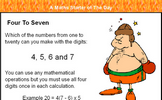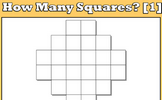# Angles in parallel lines and polygons

### Term 3 starting in week 1 :: Estimated time: 3 weeks

• Understand and use basic angles rules and notation (review)
• Investigate angles between parallel lines and the transversal
• Identify and calculate with alternate and corresponding angles
• Identify and calculate with co-interior, alternate and corresponding angles
• Solve complex problems with parallel line angles
• Construct triangles and special quadrilaterals (review)
• Investigate the properties of special quadrilaterals
• Identify and calculate with sides and angles in special quadrilaterals
• Understand and use the sum of exterior angles of any polygon
• Calculate and use the sum of the interior angles in any polygon
• Calculate missing interior angles in regular polygons

For higher-attaining pupils:

• Understand and use the properties of diagonals of quadrilaterals
• Prove simple geometric facts
• Construct an angle bisector
• Construct a perpendicular bisector of a line segment

This page should remember your ticks from one visit to the next for a period of time. It does this by using Local Storage so the information is saved only on the computer you are working on right now.

## Lesson Starters

Here are some suggestions for whole-class, projectable resources which can be used at the beginnings of each lesson in this block.

### 1st Lesson#### Letter Clue Calculations

Work out what the calculations might be from the letter clues.

### 2nd Lesson#### Four to Seven

Which of the numbers from one to twenty can you make with the digits 4, 5, 6 and 7?

### 3rd Lesson#### Double Trouble

Begin with one, double it, double it again and so on. How many numbers in this sequence can you write down before the register has been called?

### 4th Lesson### 5th Lesson#### M for Mathematics

Write down as many mathematical words as possible that begin with the letter M.

### 6th Lesson#### Half a Dozen

Six calculations to complete without a calculator.

### 7th Lesson#### How Many Squares? 1

Work out how many squares are there altogether in the given pattern then tackle the chess board.

### 8th Lesson#### Maths Crossword

A small crossword puzzle containing mathematical words.

### 9th Lesson#### Magic Square

Arrange the numbers 1 to 9 in a 3 by 3 grid to form a magic square.

Some of the Starters above are to reinforce concepts learnt, others are to introduce new ideas while others are on unrelated topics designed for retrieval practice or and opportunity to develop problem-solving skills.

White Rose ResourcesEnd of block assessments provide a quick progress check at the end of each block of learning to make sure students have understood the content covered. This Scheme of Learning was produced by White Rose Maths and is used here with permission granted on 30th June 2021.For All: